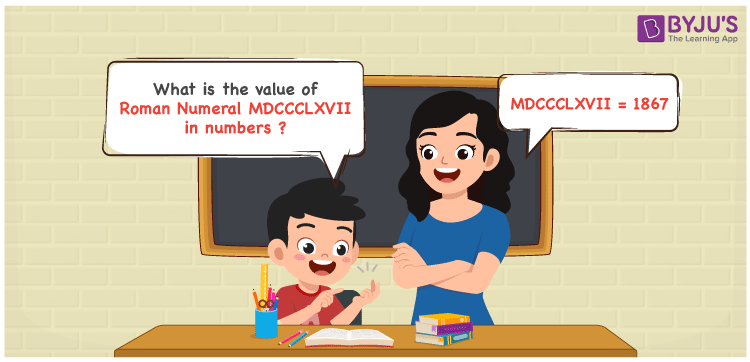Checkout JEE MAINS 2022 Question Paper Analysis : Checkout JEE MAINS 2022 Question Paper Analysis :

# MDCCCLXVII Roman Numerals

MDCCCLXVII Roman Numerals is 1867. Roman Numerals are the letters used by the ancient Romans for the representation of cardinal numbers. Access the Roman Numerals Conversion in PDF format to learn the basic rules of converting Roman letters into numbers in a comprehensive manner. Let us learn the simple tricks of representing the number form of the Roman Numeral MDCCCLXVII in this article.

 Number Roman Numeral 1867 MDCCCLXVII

## How to Write MDCCCLXVII Roman Numerals?MDCCCLXVII Roman Numerals in numbers can be written by using any of the two methods given below

First Method:

• Break the Roman Numerals into single letters
• MDCCCLXVII = M + D + C + C + C + L + X + V + I + I
• Write the numerical value of each letter and add/subtract them
• MDCCCLXVII = 1000 + 500 + 100 + 100 + 100 + 50 + 10 + 5 + 1 + 1 = 1867

Second Method:

In this method, we consider the groups of Roman Numerals for addition or subtraction

• MDCCCLXVII = M + DCCC + LX + VII = 1000 + 800 + 60 + 7 = 1867
• Therefore the numerical value of MDCCCLXVII Roman Numerals is 1867

## Video Lesson on Roman Numerals## Related Articles

Roman Numerals

Roman Numerals 1 to 50

Roman Numerals 1 to 100

Roman Numerals 1 to 1000

L Roman Numerals

Roman Numeral XXXIX

## Frequently Asked Questions on MDCCCLXVII Roman Numerals

### How do you write the numerical value of MDCCCLXVII Roman Numerals?

The numerical value of MDCCCLXVII Roman Numerals is written as 1867.

### What is the difference between 2000 and 1867 in Roman Numerals?

2000 – 1867 = 133. The number 133 in Roman Numerals is CXXXIII. Hence the difference between 2000 and 1867 in Roman Numerals is CXXXIII.

### Write the Arabic Numeral of MDCCCLXVII Roman Numeral.

1867 is the Arabic Numeral of MDCCCLXVII Roman Numeral.